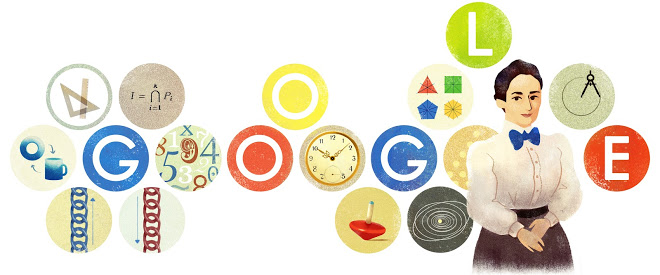#### A short note on functionals, conservation, invariance and symmetry

October 8, 2016The thing starts with invariance, conservation and symmetry

Invariant is something that is unchanged by a coordinate transformation.

Conserved is something that is unchanged by a process within a given coordinate system.

Symmetry could be considered a broader property of some ‘universe’ in which some entity of interest remains the same under a specific operation. To measure symmetry one needs to apply some transformation and see if there is a change in the object of interest.

In addition, theories of relativity are being built on entities that are supposed to be invariant among various coordinate systems.

Then the whole consideration moves on to the functionals

A functional is a ‘mathematical tool’ that takes functions and maps them into real numbers.

This is indeed the work of definite integrals, so usually functionals are expressed as definite integrals. A typical formulation is as follows:$\displaystyle J = \int_a^b L(t,x^\mu,\dot{x^\mu}) \mathrm{d} t$
where L is the Lagrangian of the functional J and the arguments in L(.) are the independent variable, the dependent variables and their derivatives. In this definition, the Lagrangian is the integrand of the functional.

A simple example of a functional is that of the ‘distance’. The distance on a path on a plane xy between points a, b, which is described by a function y=y(x), can be defined as the integral of all infinitesimal lengths ds as follows:$\displaystyle d = \int_a^b \mathrm{d}s = \int_a^b \sqrt{\mathrm{d} x^2 + \mathrm{d} y^2} = \int_a^b \sqrt{1+\dot{y}^2} \mathrm{d} x$
assuming that$\displaystyle \dot{y} \equiv \frac{\mathrm{d} y}{\mathrm{d} x}$
exists in the closed interval [a,b].

A geodesic is nothing more than the shortest such path constrained to the same surface (the plain in this example). Since we talk about a “shortest” this means we need to consider extremals. Here we find expressions like the celebrated Fermat’s principle, which requires that a trajectory’s time interval be minimum between the end-points,$\displaystyle \Delta t = \frac{1}{c} \int_{x(a)}^{x(b)} n(x,y) \sqrt{1+ \dot{y}^2} \mathrm{d}x = min$
or the equally celebrated Hamilton’s principle, which requires that a trajectory be the shortest between two times a, b,$\displaystyle J = \int_{a}^{b} (K-U) \mathrm{d}t = min$
K-U being the well known Kinetic-Potential energy difference.

Since we are dealing with functionals and need to ‘make them’ extremals, the Euler-Lagrange equation comes to play. Since,$\displaystyle J = \int_a^b L(t,x^\mu,\dot{x^\mu}) \mathrm{d} t$

the xμ(t) that make J an extremum are the solutions of the N Euler-Lagrange equations,$\displaystyle \frac{\mathrm{d}}{\mathrm{d}t} \frac{\partial L}{\partial \dot{x}^\mu} = \frac{\partial L}{\partial x^\mu}, \; \mu=1,2,\cdots,N$

Conservation laws and (corresponding) symmetries arise as consequences of the Euler-Lagrange equations either as they are, or by including momentum. If L remains unchanged by, say, a translation, the equations result a zero and momentum becomes constant, thus conserved; that’s one symmetry. On the other side, if L remains unchanged in time then the corresponding Hamiltonian becomes constant, thus energy is preserved; that’s another symmetry.

*** A reminder for the relation between the Hamiltonian H and the Lagrangian L,$\displaystyle \frac{\partial L}{\partial t}=-\dot{H}$

What Emmy Noether did in 1918 was to interconnect extremal functionals with invariance under infinitesimal transformations in her famous Theorem, which is considered to be largely influential in modern physics during the 20th century. Her theorem states in words that if a functional is extremal and it is invariant under an infinitesimal transformation then a conservation law holds.

A consequence of this theorem was the discovery of conservation laws that could not be derived from Euler-Lagrange equations, like the conservation of both energy and momentum in cases in which neither of them is separately conserved!

*** The featured image is Google’s Emmy Noether’s 133th birthday doodle.